# Search by Topic

#### Resources tagged with Cubes & cuboids similar to Mathematical Anagrams:

Filter by: Content type:
Age range:
Challenge level:

### There are 23 results

Broad Topics > 3D Geometry, Shape and Space > Cubes & cuboids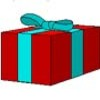##### Age 16 to 18 Challenge Level:

A box of size a cm by b cm by c cm is to be wrapped with a square piece of wrapping paper. Without cutting the paper what is the smallest square this can be?### Cubic Net

##### Age 14 to 18 Challenge Level:

This is an interactive net of a Rubik's cube. Twists of the 3D cube become mixes of the squares on the 2D net. Have a play and see how many scrambles you can undo!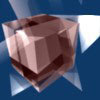### Cheese Cutting

##### Age 16 to 18 Challenge Level:

In this problem we see how many pieces we can cut a cube of cheese into using a limited number of slices. How many pieces will you be able to make?### Inside Out

##### Age 14 to 16 Challenge Level:

There are 27 small cubes in a 3 x 3 x 3 cube, 54 faces being visible at any one time. Is it possible to reorganise these cubes so that by dipping the large cube into a pot of paint three times you. . . .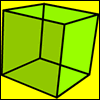### Cubic Rotations

##### Age 14 to 16 Challenge Level:

There are thirteen axes of rotational symmetry of a unit cube. Describe them all. What is the average length of the parts of the axes of symmetry which lie inside the cube?### The Spider and the Fly

##### Age 14 to 16 Challenge Level:

A spider is sitting in the middle of one of the smallest walls in a room and a fly is resting beside the window. What is the shortest distance the spider would have to crawl to catch the fly?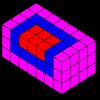### Summing Squares

##### Age 14 to 16 Challenge Level:

Discover a way to sum square numbers by building cuboids from small cubes. Can you picture how the sequence will grow?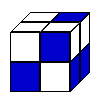### Cubic Conundrum

##### Age 7 to 16 Challenge Level:

Which of the following cubes can be made from these nets?### All Tied Up

##### Age 14 to 16 Challenge Level:

A ribbon runs around a box so that it makes a complete loop with two parallel pieces of ribbon on the top. How long will the ribbon be?### Nine Colours

##### Age 11 to 16 Challenge Level:

Can you use small coloured cubes to make a 3 by 3 by 3 cube so that each face of the bigger cube contains one of each colour?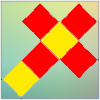### Classic Cube

##### Age 16 to 18 Challenge Level:

The net of a cube is to be cut from a sheet of card 100 cm square. What is the maximum volume cube that can be made from a single piece of card?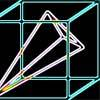### Four Points on a Cube

##### Age 16 to 18 Challenge Level:

What is the surface area of the tetrahedron with one vertex at O the vertex of a unit cube and the other vertices at the centres of the faces of the cube not containing O?### Three Cubes

##### Age 14 to 16 Challenge Level:

Can you work out the dimensions of the three cubes?### Marbles in a Box

##### Age 11 to 16 Challenge Level:

How many winning lines can you make in a three-dimensional version of noughts and crosses?### Partly Painted Cube

##### Age 14 to 16 Challenge Level:

Jo made a cube from some smaller cubes, painted some of the faces of the large cube, and then took it apart again. 45 small cubes had no paint on them at all. How many small cubes did Jo use?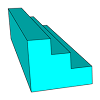### Which Solid?

##### Age 7 to 16 Challenge Level:

This task develops spatial reasoning skills. By framing and asking questions a member of the team has to find out what mathematical object they have chosen.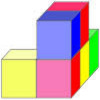### The Solid

##### Age 11 to 16 Challenge Level:

A task which depends on members of the group working collaboratively to reach a single goal.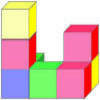### Solids

##### Age 11 to 16 Challenge Level:

A task which depends on members of the group working collaboratively to reach a single goal.### Plane to See

##### Age 16 to 18 Challenge Level:

P is the midpoint of an edge of a cube and Q divides another edge in the ratio 1 to 4. Find the ratio of the volumes of the two pieces of the cube cut by a plane through PQ and a vertex.### Classifying Solids Using Angle Deficiency

##### Age 11 to 16 Challenge Level:

Toni Beardon has chosen this article introducing a rich area for practical exploration and discovery in 3D geometry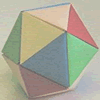### Paper Folding - Models of the Platonic Solids

##### Age 7 to 16

A description of how to make the five Platonic solids out of paper.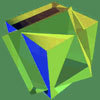### Interpenetrating Solids

##### Age 16 to 18 Challenge Level:

This problem provides training in visualisation and representation of 3D shapes. You will need to imagine rotating cubes, squashing cubes and even superimposing cubes!### Cubestick

##### Age 16 to 18 Challenge Level:

Stick some cubes together to make a cuboid. Find two of the angles by as many different methods as you can devise.